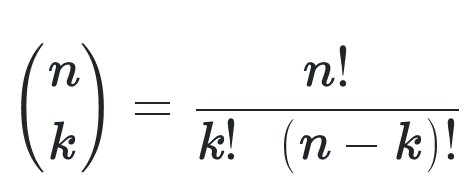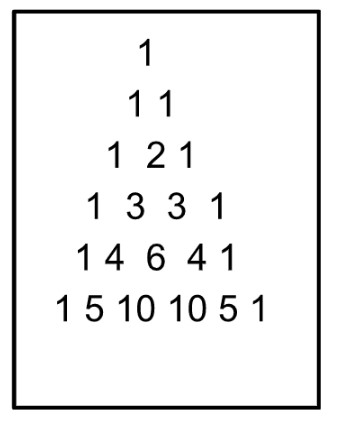# Binomial Coefficient Calculator

To use Binomial coefficient calculator, input the values of n & k, and click calculate button

Formula:
( n k ) = n! k! . (n-k)!
Random Example

Give Us Feedback

## Binomial coefficient calculator

Binomial coefficient calculator is used to calculate the number of ways to choose 'k' items from a set of 'n' items without considering the order. This concept is widely used in probability, statistics, and combinatorial problems.

## What is the binomial coefficient?

The binomial coefficient is represented as "C(n, k)" or more commonly as the combination notation "(n k)" or "nCk". It can also be expressed using the binomial theorem and Pascal's triangle. The formula for the binomial coefficient is as follows:

C(n, k) = n! / (k!(n-k)!)where

• "n" represents the total number of items
• "k" represents the number of items to choose
• "!" denotes the factorial of a number, which is the product of all positive integers up to that number.

## Uses of binomial coefficient:

Binomial coefficients are used in various fields such as probability, statistics, and computer science, as well as in combinatorial problems and algebraic expressions involving binomial expansions.

### Combinatorics

The binomial coefficient is a fundamental concept in combinatorics, which is the study of counting and arranging objects. It is used to calculate the number of ways to choose a certain number of items from a larger set, without considering the order in which they are chosen.

The binomial coefficient is essentially the formula for calculating combinations. In the context of combinatorics, the terms "binomial coefficient" and "combinations" are often used interchangeably.

### Pascal’s Triangle

Pascal's Triangle is a geometric representation of the binomial coefficients, which also showcases various mathematical properties and patterns.

Pascal's Triangle is a triangular array of numbers, where each number is the sum of the two numbers directly above it.

The triangle starts with a single "1" at the top and expands outward, with the first and last number in each row being 1. The rows and columns are indexed starting from 0.

Here's the beginning of Pascal's Triangle:The binomial coefficients appear in Pascal's Triangle in the following ways:

1. Relationship to row and column indices: The number at row n and column k in Pascal's Triangle represents the binomial coefficient C(n, k). Row numbers correspond to the total number of items in the set (n), while column numbers correspond to the number of items chosen from the set (k).
2. The sum of consecutive coefficients: The sum of any two consecutive binomial coefficients in a row is equal to the binomial coefficient in the row below and between them. Mathematically, this property can be represented as:

C(n, k) + C(n, k+1) = C(n+1, k+1)

1. Binomial theorem and expansions: The binomial coefficients in Pascal's Triangle are related to the binomial theorem, which states that the coefficients in the expansion of (a + b)^n are the binomial coefficients of the nth row.

For example, the coefficients in the expansion of (a + b)^4 are 1, 4, 6, 4, and 1, corresponding to the fourth row of Pascal's Triangle.

1. Combinatorial identity: Pascal's Triangle demonstrates the symmetry property of the binomial coefficients, which states that C(n, k) = C(n, n-k). This property is visually evident, as each row in Pascal's Triangle is symmetrical.

## How to find the binomial coefficient?

The n and k are mostly provided in every problem. You have to plug in those values in the formula. Alternatively, you can use the binomial coefficient calculator.

For example, let's say you have a set of 5 objects (n = 5) and you want to choose 2 of them (k = 2). Using the formula, you would calculate the binomial coefficient like this:

C(5, 2) = 5! / (2!(5-2)!)

= 120 / (2 * 6)

= 120 / 12

= 10

So, there are 10 ways to choose 2 items from a set of 5 without considering the order.

### Math Tools